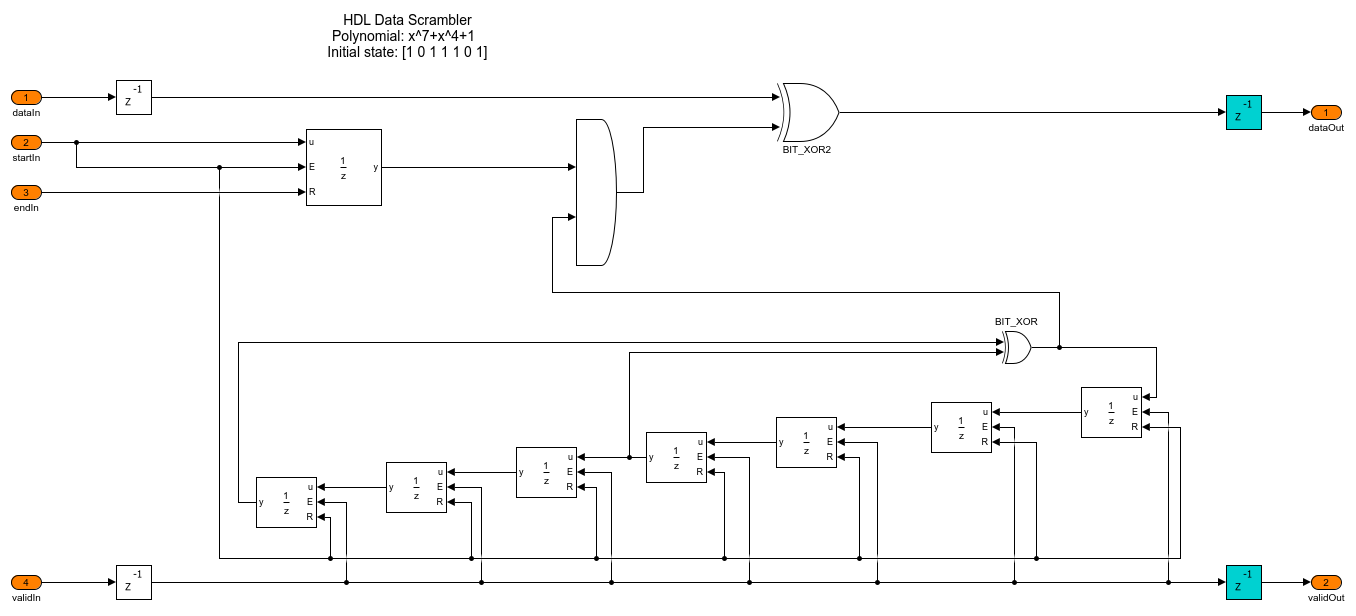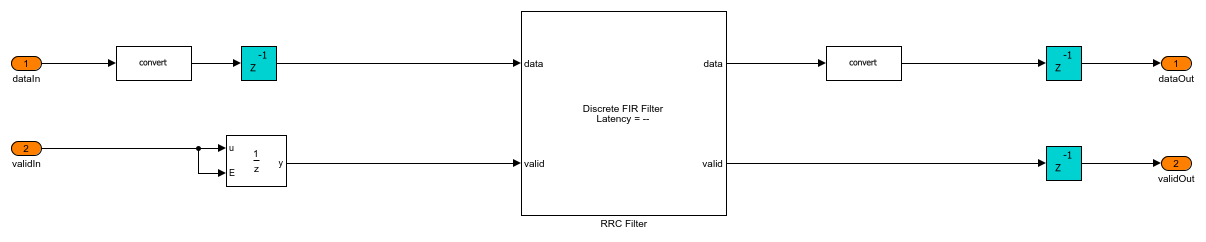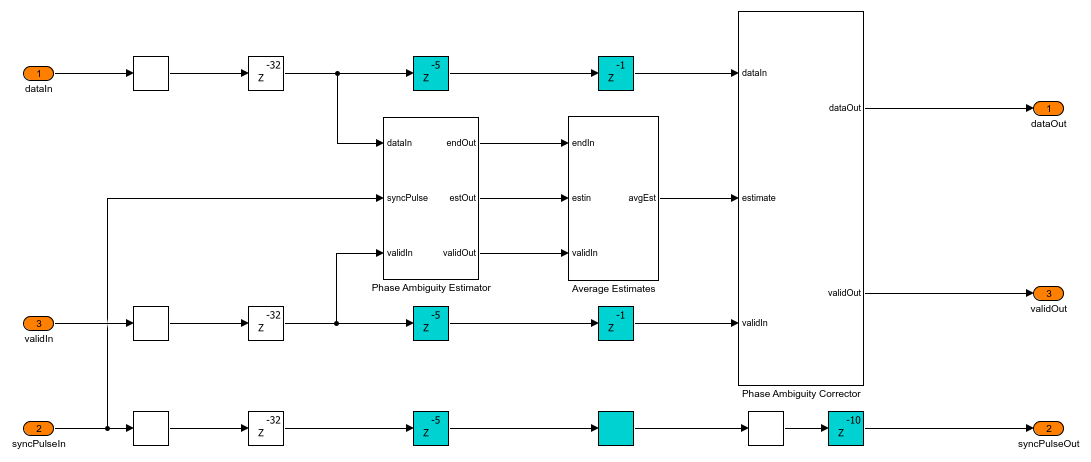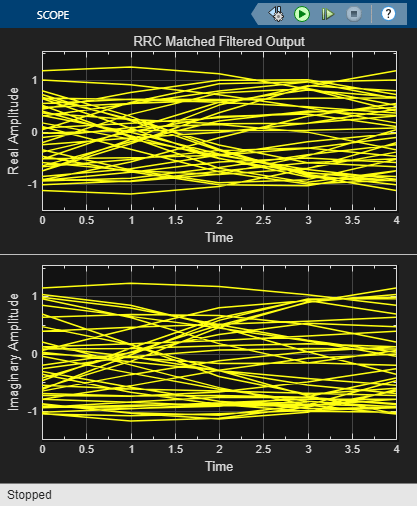# HDL QPSK Transmitter and Receiver

This example shows how to implement a QPSK transmitter and receiver in Simulink® that is optimized for HDL code generation and hardware implementation.

The model shown in this example modulates data based on quadrature phase shift keying (QPSK). The goal of this example is to model an HDL QPSK communication system that can transmit and recover information for a real-time system. The receiver implements symbol timing synchronization and carrier frequency and phase synchronization, which are essential in a single-carrier communication system.

### System Specifications

This section explains the specifications of the transmitter and receiver used in this example. The frame format is packet based. Each packet has a preamble of length 26 bits. Each bit of the 13 bit Barker sequence is repeated twice to generate a preamble sequence such that the same bit is modulated in the in phase and quadrature phase by the QPSK Modulator. The preamble sequence is followed by 2240 bits of payload data. The transmitter runs using a root raised cosine (RRC) pulse-shaping filter with a roll-off factor of 0.5, resulting in a bandwidth of 1.5 times the symbol rate and four samples per symbol (sample rate of four times the symbol rate). The RRC impulse response spans over four adjacent symbols. The bit rate is twice the symbol rate. The effective average bit rate is the bit rate times the frame efficiency. The frame efficiency is (2240/(2240+26)) = 0.9885.

The default symbol rate is set to 1.92 Mbaud, which results in a bandwidth of 1.5 times 1.92e6, which equals 2.88 MHz, and a sample rate of 4 times 1.92e6, which equals 7.68 Msps, bit rate of 2 times 1.92e6, which equals 3.84 Mbps. The effective average bit rate supported by this system is 0.9885 times 3.84e6, which equals 3.7959 Mbps. These specifications change with a change in the symbol rate.

### Model Architecture

This section explains the high-level architecture of the QPSK transmitter and receiver as in the block diagram. The QPSK transmitter samples the input at a bit rate of twice the symbol rate. The Data Generator & Packetizer collects the data bits, generates the preamble bits, and forms the packet bits. The HDL Data Scrambler scrambles the data bits of each packet to increase bit transitions and avoid long running sequences of the same bit. The QPSK Modulator modulates the packet bits to generate QPSK symbols. The RRC Transmit Filter upsamples and pulse-shapes the QPSK symbols to generate the Tx Waveform at a sample rate of four times that of the symbol rate. The QPSK receiver samples the input at the transmission rate. The Digital AGC performs gain control to the desired amplitude level of the received waveform. The RRC Receive Filter performs matched filtering on the AGC output. The Frequency and Time Synchronizer performs synchronization operations and generates QPSK symbols for each packet. The QPSK Demodulator demodulates the QPSK symbols to generate packet bits. The HDL Data Descrambler descrambles the packet data bits that stream out of the receiver.### File Structure

One Simulink model and three MATLAB® files construct this example.

• commhdlQPSKTxRx.slx — Top-level Simulink model

• commhdlQPSKTxRxParameters.m — Generates parameters for QPSK Tx and QPSK Rx required for initialization

• commhdlQPSKTxRxModelInit.m — Initializes the model commhdlQPSKTxRx.slx

• generateHelloworldMsgBits.m — Generates "Hello world xxx " message bits. xxx refers to values from 000 to 100

### System Interface

This figure shows the top-level model of the QPSK transmitter and receiver system.Transmitter Inputs

• dataIn — Input data, specified as a Boolean scalar.

• validIn — Control signal to validate the dataIn, specified as a Boolean scalar.

Transmitter Outputs

• dataOut — Output transmitted waveform, returned as 16-bit complex data at a sample rate four times that of the symbol rate.

• validOut — Control signal to validate the dataOut, returned as a Boolean scalar.

• txDiagBus — Status signal with diagnostic outputs, returned as a Bus signal.

• dataReady — Signal to indicate a ready for the input signals, returned as a Boolean scalar.

The transmitter enables the dataReady signal to indicate that it is ready to accept input bits. The transmitter constructs a packet after it accepts all the data bits corresponding to that packet. If all the data bits corresponding to that packet are not received, the transmitter generates dummy packets. For a dummy packet, the Barker sequence is not used for the preamble and the data bits are generated randomly internally. As long as the input bit rate is less than or equal to the effective bit rate, the dataReady signal remains high so that the input does not get any back pressure from dataReady.

• dataIn — Input data, specified as a 16-bit complex data with sample rate as the transmitter output.

• validIn — Control signal to validate the dataIn, specified as a Boolean scalar.

• dataOut — Decoded output data bits, returned as a Boolean scalar.

• ctrlOut — Bus signal with start, end, and valid signals, returned as a bus signal.

• rxDiagBus — Status signal with diagnostic outputs, returned as a bus signal.

### Transmitter Structure

This figure shows the top-level model of the QPSK Tx subsystem.Bit Packetizer

The Bit Packetizer subsystem consists of a Packet Controller MATLAB function, a Bits Store, and a Multiplexer subsystem. The preamble sequence is stored in an look up table (LUT) inside the Preamble Bits Store subsystem. The data bits stream into the Bits Store subsystem and are stored in a RAM inside the Data Bits Store subsystem. The Packet Controller MATLAB function reads the preamble sequence followed by the data bits stored in the RAM for each packet. The Multiplexer subsystem streamlines the preamble bits and the data bits.The Data Bits Store subsystem consists of a RAM that can store two packets. This RAM provides the flexibility to operate the transmitter with a discrete valid input. The Packet Controller MATLAB function reads data from the RAM only if the RAM contains a minimum of one packet of the data bits. The read and write logic is designed in such a way that the RAM does not overflows. When the RAM does not contain a minimum of one packet of the data bits, the transmitter generates a dummy packet. The Preamble does not use the Barker sequence for a dummy packet so that preamble detection does not detect it.HDL Data Scrambler

The HDL Data Scrambler subsystem scrambles the data bits in each packet by using the control signals generated by the Bits Generator subsystem.QPSK Modulator

The QPSK Modulator subsystem uses the QPSK Modulator Baseband block to modulate the preamble and data bits to generate QPSK symbols. It uses a gray mapping as described in this table.

 Bits Mapping ____ _________________ 00 0.70711+0.70711i 01 -0.70711+0.70711i 11 -0.70711-0.70711i 10 0.70711-0.70711iRRC Transmit Filter

The RRC Transmit Filter subsystem upsamples the input by a factor of four and uses the Discrete FIR Filter (DSP HDL Toolbox) block with an RRC impulse response to pulse-shape the transmitter waveform. The receive filter in the QPSK Receiver forms a matched filter to this transmit filter.This figure shows the top-level model of the QPSK Rx subsystem.Automatic Gain Control

As the input signal amplitude affects the symbol and carrier synchronizer phase-locked loop (PLL) performance, the Automatic Gain Control subsystem is placed ahead of them. The magnitude squared output is compared with the AGC reference to generate an amplitude error. This error is multiplied with the loop gain and passed through an integrator to calculate the required gain. The resulted gain is multiplied with the AGC input to generate the AGC output. For more information, see Chapter 9.5 of [ 1 ].The RRC Receive Filter is a Discrete FIR Filter (DSP HDL Toolbox) block with matched filter coefficients of the filter used for pulse-shaping in the transmitter. The RRC matched filtering generates an RC pulse-shaped waveform, which has zero ISI characteristics at maximum eye opening in the eye diagram of the waveform. Also, the matched filtering process maximizes the signal to noise power ratio (SNR) of the filter output.

Frequency and Time Synchronizer

The Frequency and Time Synchronizer subsystem performs symbol synchronization, coarse frequency compensation, carrier synchronization, and preamble detection for packet synchronization. It also estimates and resolves the phase ambiguity that is left uncorrected in carrier synchronization.The Symbol Synchronizer subsystem is a PLL-based implementation. It generates samples at the optimum time instant (maximum eye opening instant) as described in Chapter 8.5 of [ 1 ]. The subsystem generates one output sample for every four input samples. The Interpolation Filter subsystem implements a piecewise parabolic interpolator with a hardware resource efficient farrow structure as described in Chapter 8.4.2, and the farrow coefficients are tabulated in Table 8.4.1 (the free parameterof the coefficients is taken as 0.5) of [ 1 ]. This filter introduces fractional delays in the input waveform. The Gardner TED subsystem implements a Gardner timing error detector. The timing error detector is described in Chapter 8.4.1 of [ 1 ]. The loop filter filters the timing error and the timing error is passed on to the Interpolation Control MATLAB function block. This block implements a mod-1 decrementing counter to calculate fractional delays based on the loop filtered timing error as described in Chapter 8.4.3 of [ 1 ] to generate interpolants at optimum sampling instants. The Rate Handle subsystem selects the required interpolant indicated by the strobe. This sample corresponds to the maximum eye opening of the eye diagram before symbol synchronization.The Coarse Frequency Compensator subsystem raises the input sequence to a power of 4 in the Raise Power to 4 subsystem. This eliminates the QPSK phase mapping dependency in the input sequence but reduces the estimation range by a factor of 4. This sequence streams into the Coarse Frequency Estimator subsystem. The estimate obtained from the Coarse Frequency Estimator subsystem is divided by 4 to remove the factor 4 due to raising to power 4 and get the normalized coarse frequency estimate. This estimate drives the NCO (DSP HDL Toolbox) block to generate complex exponential phase that is conjugated and multiplied with the input sequence to correct the frequency offset.The Coarse Frequency Estimator subsystem differentially detect the input sequence and extract the complex frequency offset estimate in the input. This estimate is averaged for 2^15 consecutive estimates in the Integrator subsystem to get the final complex estimate. The Complex to Magnitude-Angle (DSP HDL Toolbox) block extracts the frequency from the complex estimate in the Extract Frequency subsystem.

The frequency estimator estimates a normalized frequency (with respect to symbol rate) range of -0.125 to 0.125 which corresponds to a frequency offset range of -240 KHz to 240 KHz for a symbol rate of 1.92 Mbaud. The estimation accuracy is such that the residual frequency offset after coarse frequency offset correction is with in the normalized frequency range of -0.0016 to 0.0016 which corresponds to a frequency offset range of -3 KHz to 3 KHz for a symbol rate of 1.92 Mbaud that the Carrier Synchronizer PLL converge.The Carrier Synchronizer subsystem is a TYPE II PLL with a sinusoidal phase error detector, which operates at a 45 degrees operating point. The phase error detector is described in Chapter 7.2.2, and the design equations are described in the Appendix C of [ 1 ]. A detailed analysis of TYPE II PLL with a zero operating point sinusoidal phase detector is described in Chapter 4 of [ 2 ]. The sign function of the phase detector in the real and imaginary parts converts all of the angles in the 4 quadrants into a first-quadrant angle (0 to 90 degrees), which creates an ambiguity of 90,180,270 degrees for second (90 to 180 degrees), third (-180 to -90 degrees) and fourth (-90 to 0 degrees) quadrant angles, respectively. The phase error is calculated as a deviation from the operating point (45 degrees) of the phase detector. The proportional plus integrator filter in the Loop Filter subsystem filters the phase error. The loop filter sets the normalized loop bandwidth (normalized by the sample rate) and the loop damping factor. The default normalized loop bandwidth is set to 0.005, and the default damping factor is set to 0.7071. The filtered error is given as a phase increment source to the Direct Digital Synthesis subsystem, which uses the NCO (DSP HDL Toolbox) block for complex exponential phase generation. The complex exponential phase is used to correct the frequency and phase of the input. A detailed analysis of direct digital synthesis is described in Chapter 9.2.2 of [ 1 ].The Preamble Detector subsystem performs continuous correlation for the input with the Barker sequence. The correlation is implemented as convolution with the reversed Barker sequence as coefficients for the Discrete FIR Filter (DSP HDL Toolbox) block, and the magnitude of the correlated output is found using the Complex to Magnitude-Angle (DSP HDL Toolbox) block inside the Correlator subsystem. The magnitude of the correlation is compared with a threshold. The Peak Search subsystem begins searching for the maximum correlation peak that exceeded the threshold for every one frame duration and records the timing offset. The Timing Adjust subsystem synchronizes packet timing based on the timing offset to generate syncPulse signal, which indicates a packet synchronized sample to the subsequent subsystem.The Phase Ambiguity Estimation and Correction subsystem works based on the unique word method for phase ambiguity resolution described in Chapter 7.7.1 of [ 1 ]. This method uses the preamble sequence as the reference sequence. The reference sequence is conjugated and multiplied with the preamble sequence in the input, and the residual phase is extracted as the phase ambiguity estimate. This estimate is used to correct the ambiguity by rotating the constellation in the opposite direction of ambiguity.The Packet Controller subsystem generates control signals for the packet boundaries.

QPSK Demodulator

The QPSK Demodulator subsystem uses the QPSK Demodulator Baseband block to demodulate the packet synchronized symbols and generate bits.HDL Data Descrambler

The HDL Data Descrambler subsystem descrambles the demodulated bits to generate the user bits. This subsystem is same as the scrambler used at the transmitter side.

### Run the Model

Run the commhdlQPSKTxRx.slx Model to simulate it. You can set custom data on the Input Data subsystem Mask and set channel configuration on the Channel subsystem Mask. The QPSKTxRxVerification.m script describes a procedure to verify the commhdlQPSKTxRx.slx model. This verification script generates a reference waveform within the script, compares the reference waveform with the transmitter output, and compares the transmitted bits with the receiver decoded user bits.

### Verification and Results

Run sim commhdlQPSKTxRx to run the model.

>> sim commhdlQPSKTxRx;

Simulation completed Running the verification script QPSK Tx: Maximum absolute symbol error: Real:1.4496e-05 Imaginary:1.4496e-05 Maximum absolute RRC output error: Real:7.8708e-05 Imaginary:7.8708e-05 QPSK Rx: Initial frames not compared : 29 Number of packets missed = 0 out of 30 Number of packets false detected = 0 out of 30 Number of bits errored = 0 out of 67200### HDL Code Generation

Pipeline registers (shown in cyan) are added throughout the model to make sure the transmitter and receiver subsystems do not have a long critical path.

To check and generate the HDL code referenced in this example, you must have the HDL Coder™ product.

To generate the HDL code for transmitter and receiver subsystems, update the models and use the following command:

 makehdl('commhdlQPSKTxRx/QPSK Tx') and makehdl('commhdlQPSKTxRx/QPSK Rx')

To generate test bench, use the following command:

 makehdltb('commhdlQPSKTxRx/QPSK Tx') and makehdltb('commhdlQPSKTxRx/QPSK Rx')

Test bench generation time depends on the simulation time.

The resulting HDL code is synthesized for the Xilinx® Zynq®-7000 ZC706 evaluation board. The post place and route resource utilization is shown in this table. The maximum frequency of operation is 276 MHz for the transmitter and 198 MHz for the receiver.

 Resources Tx Usage Rx Usage _______________ ________ ________ Slice Registers 321 10496 Slice LUT 160 7483 RAMB36 0 10 RAMB18 1 1 DSP48 18 118 

### Further Exploration

You can modify the channel conditions by tuning the variables listed in this table on the Channel subsystem mask in the commhdlQPSKTxRx.slx model and run the model.

 Variable Name Description ______________________ _______________________________________________________________________________________ dataBits Data bits to the transmitter Rsym Symbol rate specified in symbols per second fractionalTimingOffset Normalized timing phase offset specified in the range >= 0 and < 1 timingFrequencyOffset Timing frequency offset specified in PPM EbN0dB Energy per information bit to single sided noise power spectral density specified in dB CFO Carrier frequency offset specified in Hz CPO Carrier phase offset specified in degrees 

### References

1. Michael Rice, Digital Communications - A Discrete-Time Approach, Prentice Hall, April 2008.

2. Floyd M.Gardner, Phaselock Techniques, Third Edition, John Wiley & Sons, Inc., 2005